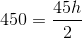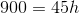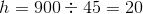# Intermediate Geometry : How to find the height of an acute / obtuse triangle

## Example Questions

### Example Question #1 : How To Find The Height Of An Acute / Obtuse Triangle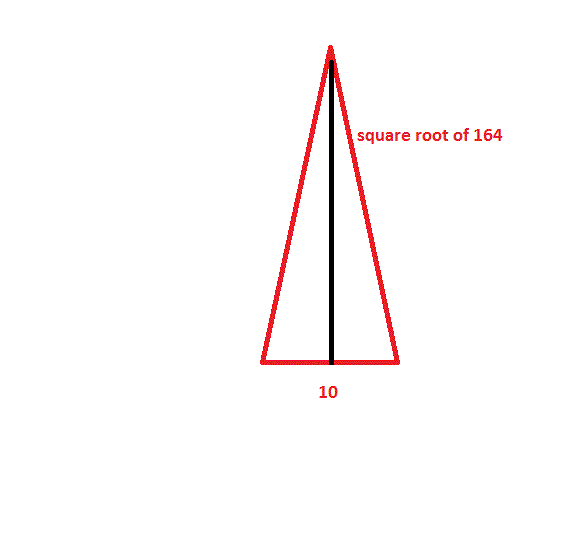Find the height of the triangle shown above.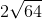Explanation:

Use the Pythagorean Theorem to find the height of this triangle:, where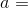the height of the triangle.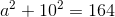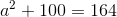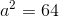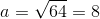### Example Question #2 : How To Find The Height Of An Acute / Obtuse Triangle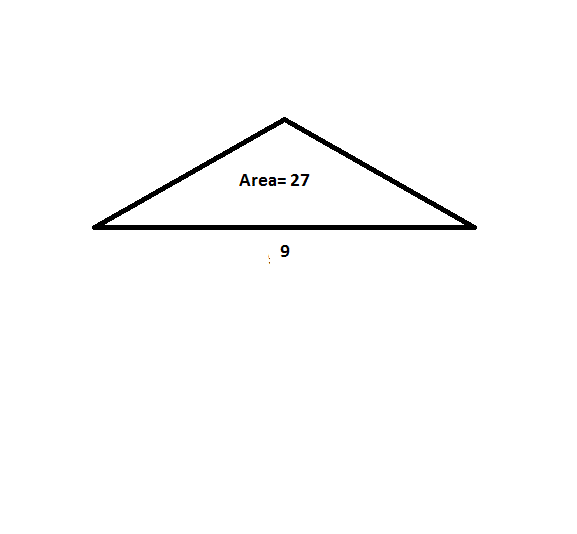Find the height of the obtuse triangle shown above.Explanation:

To solve this solution, first work backwards using the formula: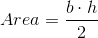Plugging in the given values we are able to solve for the height.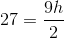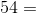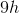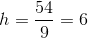### Example Question #3 : How To Find The Height Of An Acute / Obtuse Triangle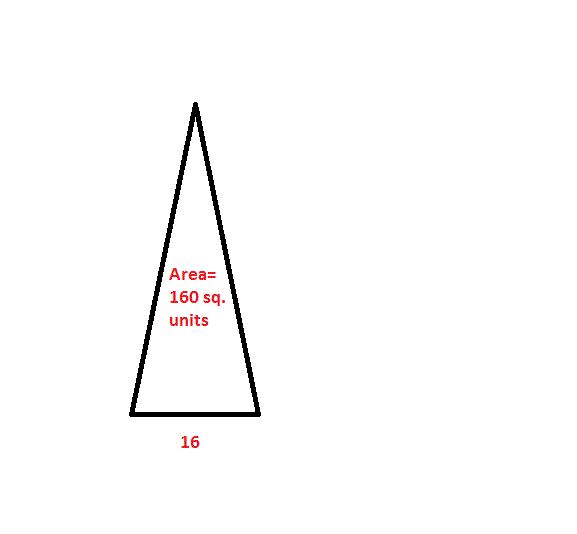Find the height of the acute triangle shown above.Explanation:

To solve this solution, work backwards using the formula:Plugging in the given values we are able to solve for the height.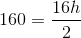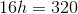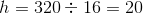### Example Question #4 : How To Find The Height Of An Acute / Obtuse Triangle

A triangle has a base ofand an area ofsquare units. Find the height of the triangle.Explanation:

To solve this solution, work backwards using the formula:Plugging in the given values we are able to solve for the height.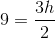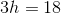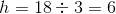### Example Question #5 : How To Find The Height Of An Acute / Obtuse Triangle

An obtuse triangle has a base ofand an area of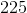square units. Find the height of the triangle.Explanation:

To solve this solution, work backwards using the formula:Plugging in the given values we are able to solve for the height.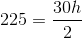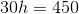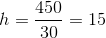### Example Question #6 : How To Find The Height Of An Acute / Obtuse Triangle

An obtuse triangle has a base ofand an area of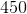square units. Find the height of the triangle.Explanation:

To solve this solution, first work backwards using the formula:Plugging in the given values we are able to solve for the height.